# Diodes

A diode is like a one-way road for electric current. Let's have a look at this simple semiconductor components.

## Diodes

In the last tutorial we looked at what's at the heart of almost all semiconductor devices: the p-n junction. We looked at the processes occurring at the junction which make it a one-way road for the electric current. A barrier forms between the differently doped regions of the silicon. In forward bias configuration the barrier shrinks and if the forward voltage is exceeded a current can flow through the p-n junction. In reverse direction the barrier size increases, no current can flow except for a small leakage current.

A diode is an electric component with two terminals that only conducts current in one direction. In the early days, this was done by using vacuum tubes. Today we use semiconductor diodes, which can achieve the same unidirectional conductance behavior using a p-n junction. As this behavior allows rectifying alternating current we usually speak of rectification instead of a unidirectional conductance behavior. We will look at the use of diodes in rectifiers and other applications in some of the upcoming tutorials, but let's focus on the basics first.

In the top left of the image below you can see the symbol used for diodes in schematics. It consists of a triangle that points into the direction of the conventional current flow. The diode only conducts if we connect + to the p-type doped region and - to the n-type doped region of the diode. If the diode is forward biased, the lamp in the shown example circuit lights up. In case of a reverse biased diode no current can flow and the lamp stays dark.The diode terminals are usually referred to as anode and cathode. These terms are commonly used in electrochemistry and also for vacuum tubes. As this terms might be unfamiliar to you let me explain them quickly. The anode is always the electrode that accepts electrons from the surrounding medium. In case of a diode this matches the p-type doped side which receives the electrons crossing the p-n junction. The cathode is the electrode that provides these electrons. In case of our semiconductor diode this is the n-type doped region of the diode. To differentiate between the anode and the cathode of a diode the cathode is usually a marked with a bar. For light emitting diodes it is common mark the cathode with a flat spot in the plastic case.

## Types of Diodes

Let's have a look at the different types of diodes. Here are some of the most important ones:

• Signal diodes
• Rectifier diodes
• Schottky diodes
• Zener and avalanche diodes
• Photodiodes and solar cells
• Light emitting diodes (LEDs)

The differences between the types lay in the construction, the materials that are used to build them and the doping level of the semiconductor material. In this tutorial we will concentrate on the first three types which are the ones that one typically thinks of when speaking about the diodes.

The difference between the first two types is mainly the size of the p-n junction. The differences determine the electrical characteristics of these diodes and thus the use cases they are suitable for. Schottky diodes are a bit more special, but we will get to that later. Let's first have a look at the parameters that define a diode. This will help us to understand the differences between signal and rectifier diodes and the important role of schottky diodes.

## Diode Characteristics

The image below shows an example for the I-V characteristics of typical p-n junction diodes. Let's have a look at the important parameters that can be used to describe the behavior of a diode.### Forward Voltage

The first important parameter is the so-called forward voltage $$V_F$$. As we learned in the tutorial on the p-n junction this is the voltage at which a forward biased diodes starts to conduct. There is room for interpretation, here, which current has to flow before the diode is called conducting. There is almost always a very small leakage current flowing, even in the reverse direction. This is not what is meant by the diode conducts. The exact test condition should be given in the diodes datasheet. A lot of DMM that have a diode tester give you the voltage that is required for 1 mA of current to flow. Datasheets of smaller diodes usually specify the forward voltage to be the voltage where 10 mA of current flow through the diodes. For bigger diodes it is even possible that the forward voltage is given for a current of 1 A or more. The forward voltage is not constant and depends on the forward current and the temperature. For details and for a comparison between different diodes one should use the more detailed graphs from the diodes datasheet.

In general the forward voltages of diodes are pretty similar to each other as they are mainly determined is the semiconductor material used in the diode. For silicon the forward voltage is usually around 0.6 V - 0.7 V for 10 mA of current. The older germanium diodes have a lower forward voltage of 0.3 V at 10 mA. Such a germanium diode is shown in the picture below.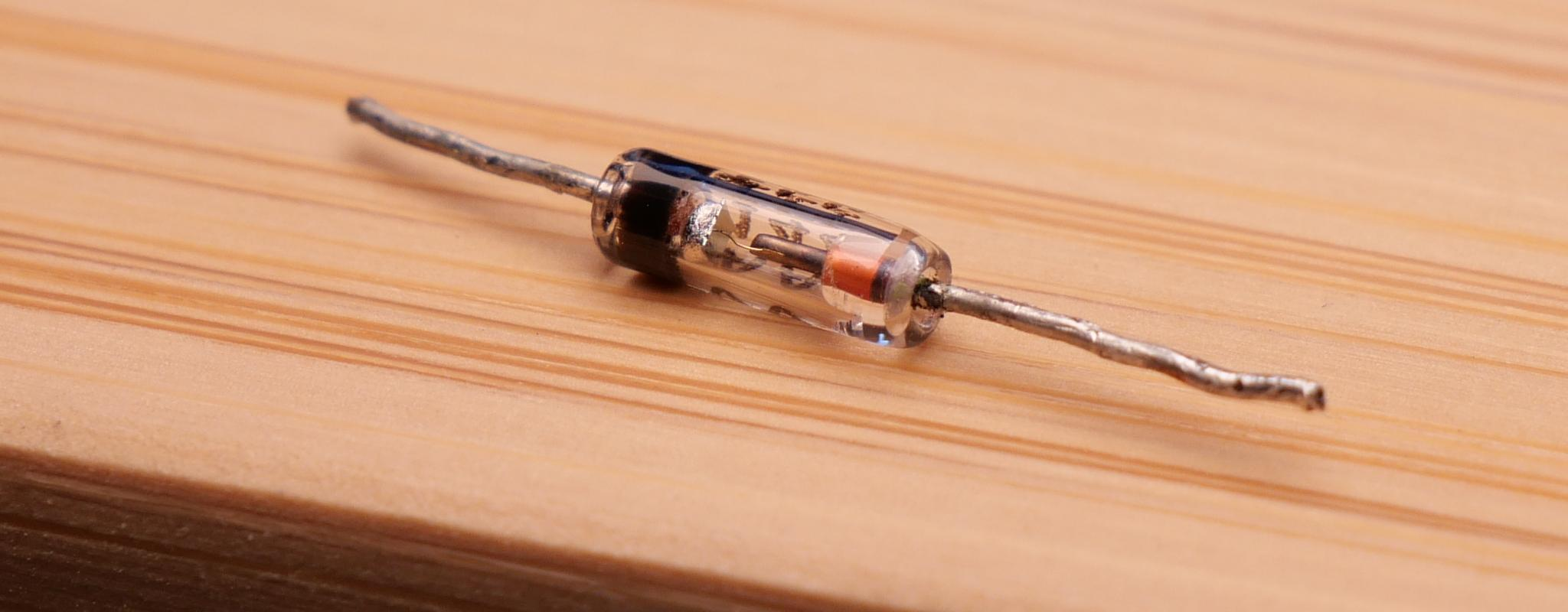Germanium diodes are not used anymore as they have the drawback that their behavior has a high temperature dependence. They were used for a long time especially in high frequency applications. Today they have been vastly replaced schottky diodes. Schottky diodes don't feature a p-n junction, but a metal-semiconductor junction instead. Their forward voltage is only 0.2 V - 0.3 V for 10 mA of current. This is one of the points that make them good replacements for germanium diodes.

### Reverse Leakage Current

As discussed in the tutorial on the p-n junction and shown in the I-V characteristics a small leakage current can flow even if the diode is reverse biased. For normal diodes this leakage current very small. In datasheets you can usually find the exact value given as reverse current $$I_R$$. A typical value is a few microamperes.

### Absolute Maximum Ratings

Similarly important as the forward voltage are the maximum ratings. The maximum forward continuous current $$I_F$$ specifies the maximum current that can flow through the diode for a longer time without damaging the p-n junction. The bigger the junction area is the higher the maximum current can be. It is important to also look at another tightly connected parameter: the maximum power dissipation. The diode heats up when a current flows through it. The total power dissipation $$P_{tot}$$ of a diode can be calculated by multiplying the forward current with the forward voltage:
$$P_{tot} = V_F \cdot I_F$$

To calculate this correctly, you have to look at the graphs given in the datasheet as the forward voltage is dependent on the current to a certain degree. This is why for high current diodes the forward voltage is given for a current of 1 A. It allows for a more realistic calculation of the power dissipation when just using the given value and not looking at the graphs. The maximum power dissipation should not be exceeded otherwise you risk a thermal destruction of the p-n junction. There are other parameters like the ambient temperature and air movement that affect how good the heat spreads and is dissipated. To make it possible take this into account in calculations there are additional thermal characteristics given in the datasheet. You probably don't want to go down this rabbit hole and do all the math for simple projects. My advice is to just plan with high enough safety margin.

If we look at I-V curve, we can see that there is another important parameter: the breakdown voltage $$V_{BR}$$. It specifies how high the applied reverse voltage can be before a breakdown occurs. In low voltage application the breakdown voltage usually doesn't matter, you won't exceed it anyway. For circuits with mains voltage like rectifiers it becomes important, however. Needless to say, that you shall not experiment which mains voltage if your are not a trained professional. I won't do this either. If we look at avalanche and zener diodes, however, the breakdown voltage becomes important once again, because they are specifically designed to have low breakdown voltages and to be used in reverse bias configuration. The reverse voltage $$V_R$$ specifies the voltage drop over the diode when operated in reverse bias configuration above the breakdown voltage. For normal didoes the reverse voltage is a lot higher than the forward voltage. This makes it really easy to exceed the maximum power dissipation and fry the diode even at small reverse currents.

### Switching Behavior

If diodes are used in AC circuits or circuits with a high switching speed the switching behavior of diodes becomes important too. I won't go into the details here, but for high frequency applications a low junction capacitance $$C_j$$ is required. The junction capacitance increases with the junction size. More important than the junction capacitance is the so-called reverse recovery time $$t_{rr}$$ which also increases with the junction size. If the direction of the applied voltage changes from forward to backward bias it takes some time until the current flow stops. The reason for this is the way the charge transport at the junction works. There are still some injected minority charge carriers left that did not yet recombine and cause the current flow to continue for a limited period of time. For diodes with a bigger p-n junction this can take over 1 µs. The reverse recovery time limits the maximum frequency for which the diode can be used without causing high power losses.

## Signal Diodes

Now, that we know the basic characteristics of diodes, let's look at the first type of diode: the signal diode. Signal didoes are sometimes also called switching diodes. They are designed for circuits with low currents, but high switching speeds. As shown in the picture below they are relatively small and often come in a glass enclosure. The 1N4448 diode shown in the picture below is an example for a fast switching diode. The maximum forward current of signal diodes is typically in the area of a few hundred milliamperes and the maximum power dissipation is usually under a watt.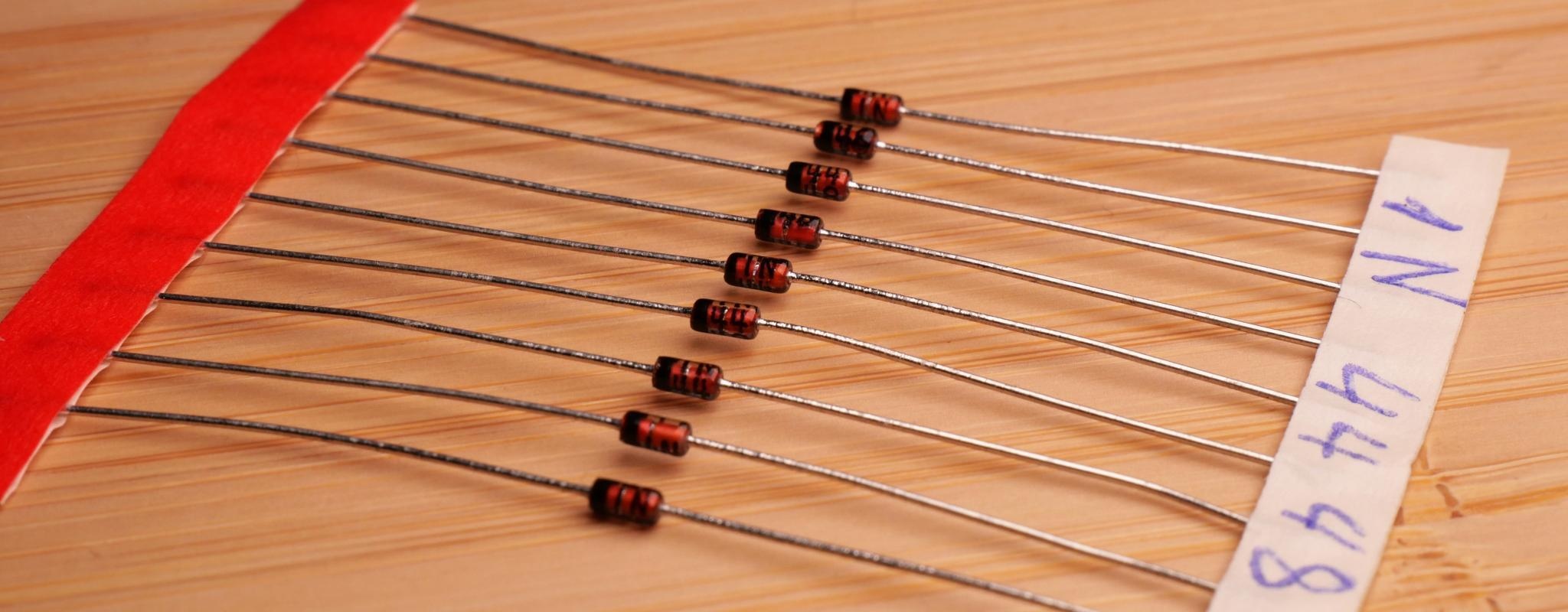In the picture below, you can see that there is only a small plate of doped semiconductor material in these diodes. While this limits the maximum current, it allows the diode to have a low junction capacitance and a low reverse recovery time of only a few nanoseconds.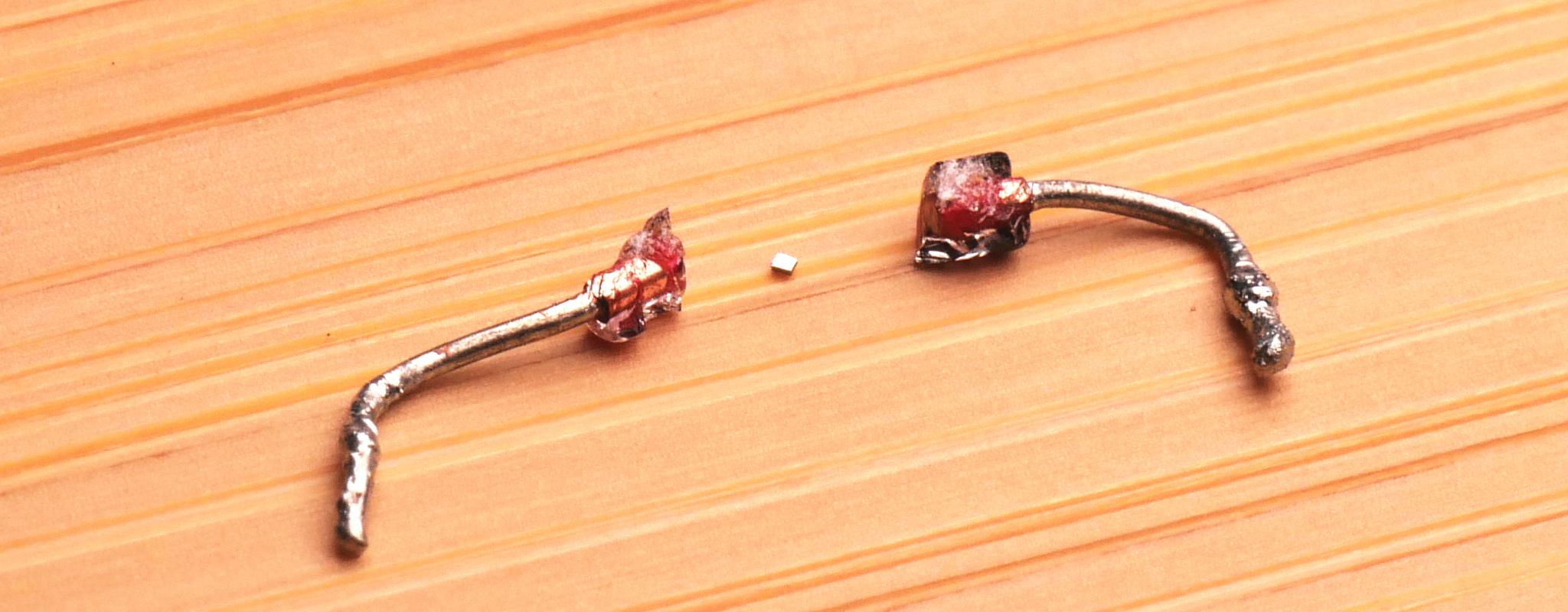Signal diodes often have a breakdown voltage in the area of around 100 V. They are therefore not suited for mains voltage rectification. The reverse leakage current is in the micro- or nanoampere range.

## Rectifier Diodes

Rectifier diodes are another popular diode type. Basically, they are just normal diodes like signal diodes, but rectifier diodes usually feature a bigger p-n junction and are therefore suitable higher forward currents. They are commonly used for mains voltage rectification or polarity protection circuits. The picture below shows a 1N4007 rectifier diode. This diode allows for a current of up to 1 A. This is still a quite small diode, but rectifier diodes for much higher currents do exist. A rectifier diode for mains voltage needs to have a high breakdown voltage. For the 1N4007 diode the breakdown voltage is 1000 V. Of course there are rectifier diodes for low voltage applications too where high breakdown voltages are not required.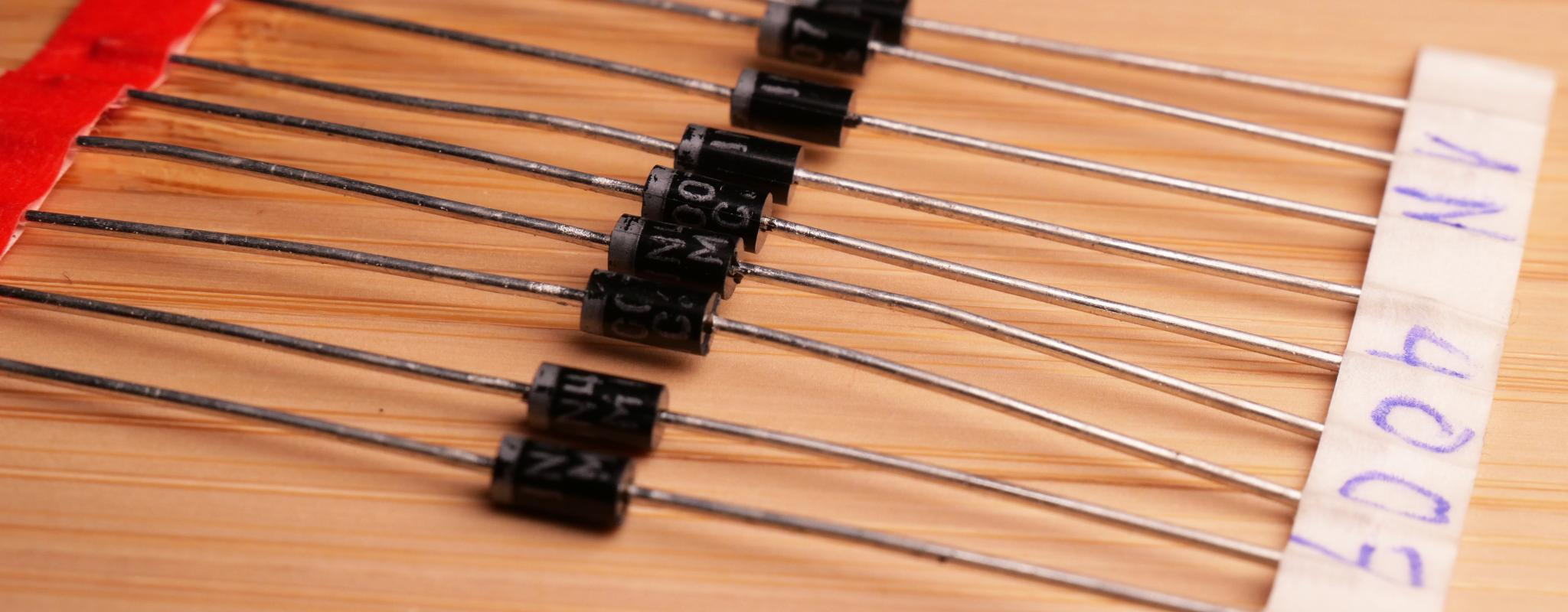In the picture below you can see the cross section of an old 1N5406 rectifier diode. This diode has a much larger p-n junction area and quite massive metal contacts. It can handle up to 3 A of current. The drawback is a higher junction capacitance and a reverse recovery time above one micro second. Such diodes are not suited for high switching frequencies. The leakage current is a bit higher due to the larger p-n junction, but still in the microampere range.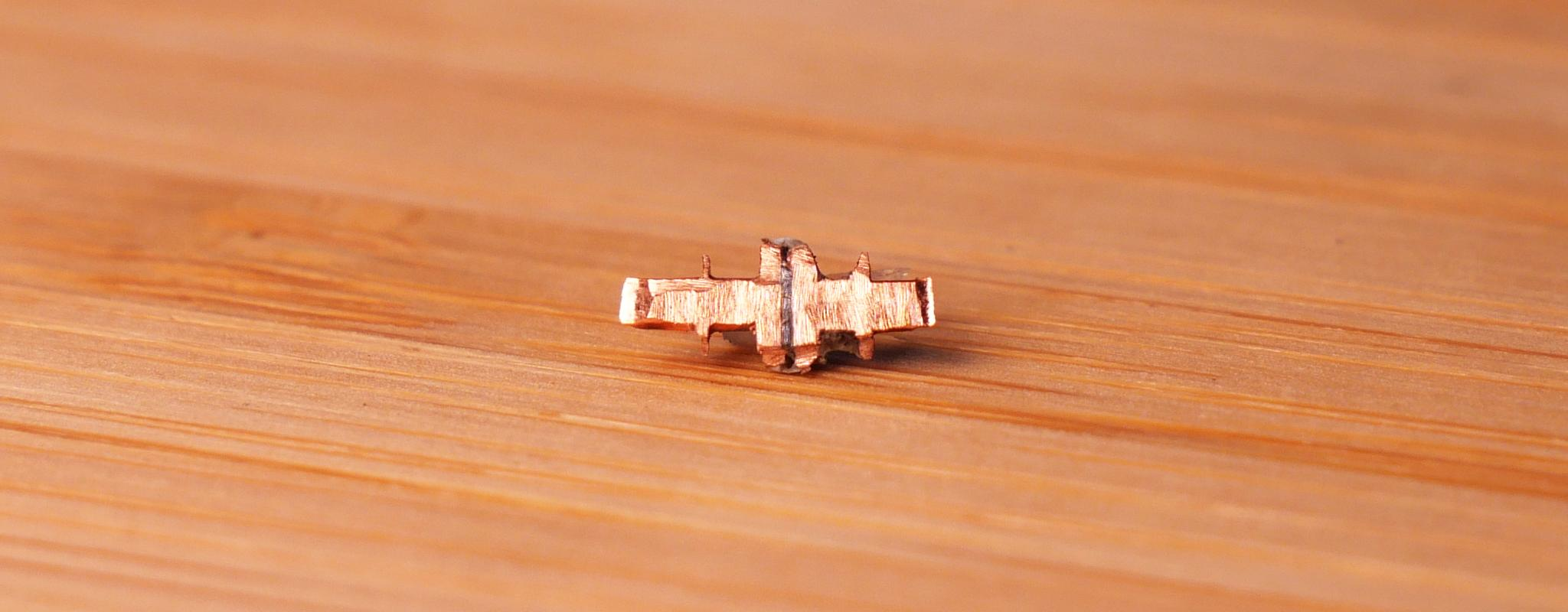## Schottky Diodes

Let's finish up with a special diode type: the schottky diode. A schottky diode is not a classical p-n junction diode, but one with a metal-semiconductor junction instead. To mark the difference, a separate symbol for schottky diodes is used in schematics.Most schottky diodes use n-type doped silicon as semiconductor material. The electrons diffuse from the n-type doped region in to the metal. The n-type doped semiconductor region becomes positively charged in proximity to the junction and the metal next to the junction becomes negatively charged. A barrier builds up just like the one at the p-n junction.

Similar to the p-n junction the barrier size increases if a reverse bias is applied and decreases if a forward bias is applied. The forward voltage needed to make the metal-semiconductor junction conductive is a lot lower, however. It is only 0.2 V - 0.3 V at a current of 10 mA. The lower voltage drop over the diode implies a reduced power dissipation as well. A lower voltage drop is also advantageous for circuits with low voltages.

There is another important difference, which makes the schottky diode suited for high frequency applications. To understand this difference we have to look at the conduction process at the metal-semiconductor junction. At a p-n junction a processes called minority charge carrier injection occurs. The charges that cross the junction are the minority charge carrier in the other region and recombine after crossing the junction. The slow recombination of the injected minority charge carriers causes the high reverse recovery time of normal p-n junction diodes. This is different for schottky diodes. When the electrons from the n-type dope region move across the metal-semiconductor junction, they act as majority charge carriers in the metal region too. In consequence there is no long reverse recovery time. This makes schottky diodes fast switching diodes. The remaining limiting factor is the junction capacitance.

Of course schottky diodes also have disadvantages. The first is the relatively high reverse leakage current with is often in the range of several milliamperes instead of micro amperes. The second is that silicon schottky diodes have a low breakdown voltage. It is usually below 100 V. This means normal schottky diodes are unsuitable for mains voltage rectification. There are also silicon carbide schottky diodes that feature higher breakdown voltage, but also don't have the advantage of the low forward voltage.

By the way, you might be wondering how diodes with metal leads work, if a barrier builds up at the junction between the semiconductor material and the metal contacts. This is indeed an issue that needs to be solved in practice. A metal-semiconductor junction without the rectifying properties of schottky diodes is needed. This is called an ohmic contact. It's possible to create one by highly doping the silicon next to the metal contact. The barrier width decreases continuously with the doping level and the barrier effect becomes finally neglectable.